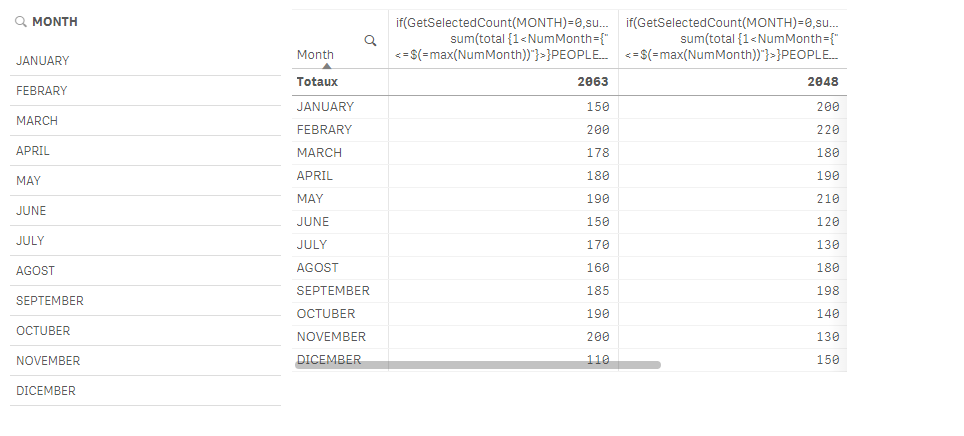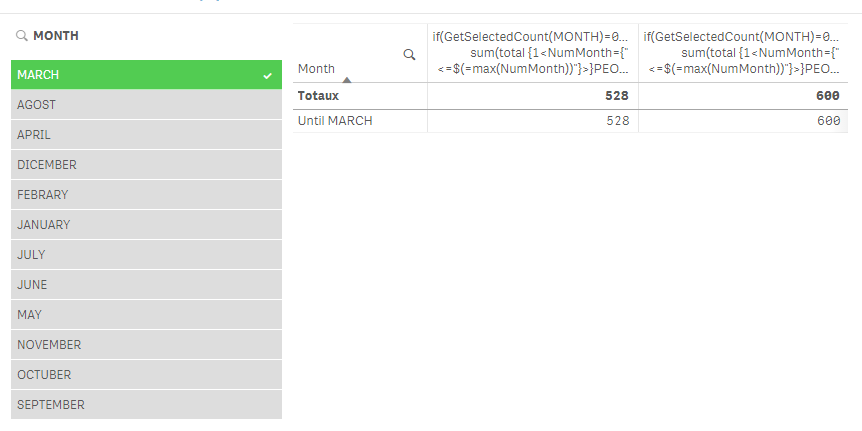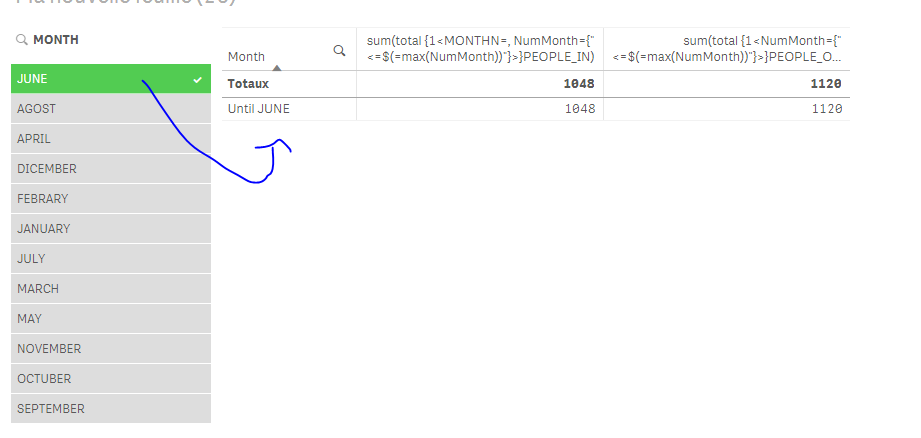New to Qlik Sense

Discussion board where members can get started with Qlik Sense.

Announcements
QlikWorld 2020: Join us May 11 - 14, 2020 in Phoenix, AZ. Register early and save \$400. Learn More
New Contributor III

How to sum range of values

Hi everybody,

I need your help with this issue:

I just trying to consolidate the values from January to Month-N (N= someone month) as a filter, because I need to reflect the total in a pivot table. Let me give an example with this table:

MONTH= Dimension

PEOPLE_IN: Measure

PEOPLE_OUT: Measure

MONTHPEOPLE_INPEOPLE_OUT
JANUARY150200
FEBRARY200220
MARCH178180
APRIL180190
MAY190210
JUNE150120
JULY170130
AGOST160180
SEPTEMBER185198
OCTUBER190140
NOVEMBER200130
DICEMBER110150

If I select January the table show it only the values from January, but if I select March the table should show me the total value from Jan to March (Jan+Feb+March) at the same time the filter should select all months back.

Filter: Jan

Select Jan

MONTHPEOPLE_INPEOPLE_OUT
JANUARY150200

Filter: Jan+Feb+March

Select Jan to March

MONTHPEOPLE_INPEOPLE_OUT
JANUARY528600
FEBRARY528600
MARCH528600

I believe that Sum(Total.....) might be a possible but really I don't know how used and how to do this.

I hope you can help me.

PD. Sorry if my english have some mistakes...

1 Solution

Accepted SolutionsPartner

Re: How to sum range of values

then change the expression as follow:

if(GetSelectedCount(MONTH)=0,sum(PEOPLE_IN), sum(total {1<NumMonth={"<=\$(=max(NumMonth))"}>}PEOPLE_IN))

if(GetSelectedCount(MONTH)=0,sum(PEOPLE_OUT), sum(total {1<NumMonth={"<=\$(=max(NumMonth))"}>}PEOPLE_OUT))16 RepliesPartner

Re: How to sum range of values

1)create a numeric month in ur script (01,02,03...)

if u have a date field: do date(dateField,'MM') as NumMonth

then, in the expression; in the table, instead of :

sum(YourMeasure)

replace it by:

sum(total {<NumMonth={"<=\$(=max(NumMonth))"}>}YourMeasure)MVP

Re: How to sum range of values

Maybe an AsOf table in your data model might help you achieve what you want:

The As-Of TablePartner

Re: How to sum range of values

But he's not cumulating here Stefan to use the as-of table as it is? no?

New Contributor III

Re: How to sum range of values

Hi guys,

Thanks for answer me. I going to check the options and I'll report about it.

Thanks.

Contributor II

Re: How to sum range of values

Hi,

Is this what you expect as your output ?

MONTHPEOPLE_INPEOPLE_OUT
JANUARY528200
FEBRARY528420
MARCH528600
Contributor II

Re: How to sum range of values

sorry, please ignore the one sent earlier and consider this.

Is this what you expect as your output ?

MONTHPEOPLE_INPEOPLE_OUT
JANUARY150200
FEBRARY300420
MARCH528600Partner

Re: How to sum range of values

Example:

1) I created a NumMonth field in the script (01 02 ...)

2) I add a filter : MONTH

3) Create a table:

Dimension: =if(GetSelectedCount( MONTH)=0,MONTH, 'Until '&MONTH)

Measure 1 : sum(total {1<NumMonth={"<=\$(=max(NumMonth))"}>}PEOPLE_IN)

Measure 2 : sum(total {1<NumMonth={"<=\$(=max(NumMonth))"}>}PEOPLE_OUT)

Result :

If I don't make any selection:If I select a MONTH:Contributor II

Re: How to sum range of values

Create a field in the script as below.

Num(Month(Date#(Month,'MMM'))) as Num_Month

People In Expression : RANGESUM(above(sum({<Num_Month = {'>=\$(1)<=\$(=max(Num_Month))'}>}PEOPLE_IN), 0, RowNo()))

People out Expression : RANGESUM(above(sum({<Num_Month = {'>=\$(1)<=\$(=max(Num_Month))'}>}PEOPLE_OUT), 0, RowNo()))

Contributor II

Re: How to sum range of values

This solution will give you the below result, if that is what you're looking for :

MONTHPEOPLE_INPEOPLE_OUT
JANUARY150200
FEBRARY300420
MARCH528600